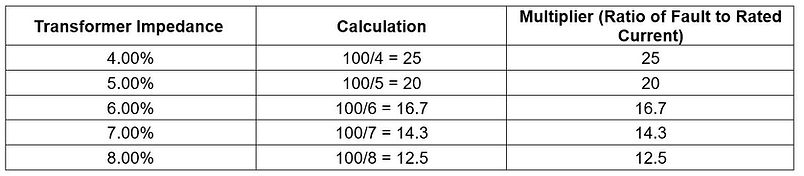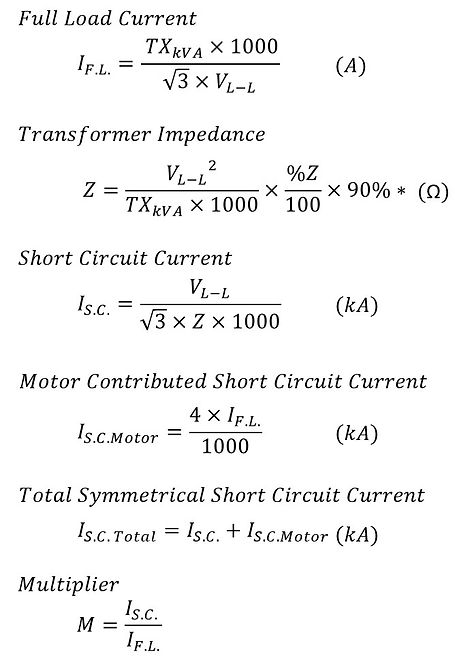Transformer Short Circuit Calculator

Short Circuits, also known as faults, can occur in electrical power distribution systems. When a fault occurs on the load side of a transformer, fault current, or short circuit current, flows through the transformer. Transformers are designed to withstand these fault currents, which are significantly higher than the rated current.

It is worth noting that transformer impedance is one of the limiting factors for the short circuit current of transformer at the secondary coil. The higher the transformer impedance (% Z), the lower the ratio of fault to rated current. In other words, a Transformer with higher %Z better limits the flow of fault current.Short Circuit Current of a Transformer is calculated using the formula as shown. Note that UL 1561 transformer has a ±10% of impedance tolerance. For high end worst case, we assume a lower transformer impedance by multiplying a 90% safety factor as shown below*.Where

IF.L.          = Full Load Current (A)

TXKVA     = Transformer Rating (kVA)

VL-L         = Phase Voltage

Z              = Transformer Impedance,

%Z           = Transformer % Impedance

IS.C.         = Short Circuit Current (kA)

M             = Multiplier, or ratio of Short Circuit Current to Full Load Current

To compute the short circuit current at a distance, L feet away from the first fault current, I S.C. calculated above, over a conductor of C-Values, C and of N number per phase, factor f and Multiplier M can be derived from the formulae below.A downloadable Excel File for Transformer Short Circuit Current Calculation, and estimated Short Circuit Current at X2, is available below:

Transformer Short Circuit Current Calculator (Excel)